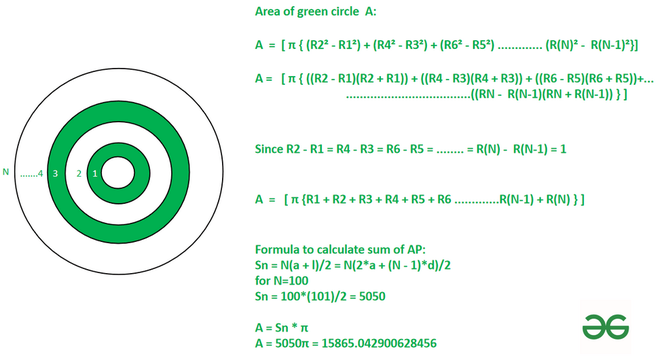Open in App
Not now

# Sum of area of alternative concentric circle with radius 1,2,3,4……….N

• Last Updated : 20 Jan, 2022

Given that Concentric circle of radii 1,2,3,4……….N cm are drawn. The interior of the smallest circle is colored white and angular regions are colored alternately green and white so that no two adjacent regions are of the same color. The task is to find the total area of the green region in sq cm.

Examples:

Input: N=100
Output: 15865.042900628456Input: N=10
Output: 172.78759594743863

Approach: Area of the first green region would be-

[π(R2²- R1²)]

Similarly, the area of all alternative green circles would be-

[π(R4²- R3²)], [π(R6²- R5²)]…..

The total area of the green region is the sum of the area of the green region is-

A  =  [ π { (R2² – R1²) + (R4² – R3²) + (R6² – R5²) …………. (R(N)² –  R(N-1)²}]

A =   [ π { ((R2 – R1)(R2 + R1)) + ((R4 – R3)(R4 + R3)) + ((R6 – R5)(R6 + R5)) …………. ((RN –  R(N – 1)(RN + R(N – 1)) } ]

Since the difference between the radius of 2 concentric circles is only 1 cm so R(N) – R(N – 1) = 1. Hence,

A  =   [ π {R1 + R2 + R3 + R4 + R5 + R6 ………….R(N-1) + R(N) } ]

(R1 + R2 + R3 + R4 + R5 + R6 ………….R(N-1) + R(N) forms an arithmetic progression so we have to find the sum of arithmetic progression with starting radius as 1 and last radius as N with a common difference of 1.

Sum of A.P. is-

SN = N * (2 * a +(N – 1) * d) / 2

or

SN = N * (a + l) / 2

Thus, the area of the green region is-

A = Sn * π

Below is the implementation of the above approach:

## C++

 `// C++ code for the above approach` `#include ` `using` `namespace` `std;`   `// Function to calculate area` `// of concentric circles` `double` `area(``int` `N, ``int` `a, ``int` `l)` `{`   `    ``// Formula to find sum of` `    ``// Arithmetic Progression` `    ``double` `S = N * (a + l) / 2;`   `    ``// return area` `    ``return` `S * M_PI;` `}`   `// Driver code` `int` `main()` `{` `  `  `    ``// N is number of concentric circles` `    ``int` `N = 100;`   `    ``// Radius of interior circle` `    ``int` `a = 1;`   `    ``// l radius of exterior circle` `    ``int` `l = N;`   `    ``// r is result` `    ``double` `r = area(N, a, l);`   `    ``// Print result` `    ``cout << fixed << setprecision(12) << r;` `}`   `// This code is contributed by Samim Hossain Mondal.`

## Java

 `// Java code for the above approach` `class` `GFG` `{` `  `  `// Function to calculate area` `// of concentric circles` `static` `double` `area(``int` `N, ``int` `a, ``int` `l)` `{`   `    ``// Formula to find sum of` `    ``// Arithmetic Progression` `    ``double` `S = N * (a + l) / ``2``;`   `    ``// return area` `    ``return` `S * Math.PI;` `}`   `// Driver code` `public` `static` `void` `main(String args[])` `{` `  `  `    ``// N is number of concentric circles` `    ``int` `N = ``100``;`   `    ``// Radius of interior circle` `    ``int` `a = ``1``;`   `    ``// l radius of exterior circle` `    ``int` `l = N;`   `    ``// r is result` `    ``double` `r = area(N, a, l);`   `    ``// Print result` `    ``System.out.println(r);` `}` `}`   `// This code is contributed by gfgking`

## Python3

 `# import pi from math module` `from` `math ``import` `pi`   `# Function to calculate area ` `# of concentric circles` `def` `area(N,a):` `      `  `    ``# Formula to find sum of ` `    ``# Arithmetic Progression` `    ``S ``=` `N ``*` `(a ``+` `l) ``/` `2` `    `  `    ``# return area` `    ``return` `S ``*` `pi`   `# N is number of concentric circles` `N ``=` `100`   `# Radius of interior circle` `a ``=` `1`   `# l radius of exterior circle` `l ``=` `N`   `# r is result ` `r ``=` `area(N, a)`   `# Print result` `print``(r)`

## C#

 `// C# program for the above approach` `using` `System;` `class` `GFG` `{`   `  ``// Function to calculate area` `  ``// of concentric circles` `  ``static` `double` `area(``int` `N, ``int` `a, ``int` `l)` `  ``{`   `    ``// Formula to find sum of` `    ``// Arithmetic Progression` `    ``double` `S = N * (a + l) / 2;`   `    ``// return area` `    ``return` `S * Math.PI;` `  ``}`   `  ``// Driver Code:` `  ``public` `static` `void` `Main()` `  ``{` `    ``// N is number of concentric circles` `    ``int` `N = 100;`   `    ``// Radius of interior circle` `    ``int` `a = 1;`   `    ``// l radius of exterior circle` `    ``int` `l = N;`   `    ``// r is result` `    ``double` `r = area(N, a, l);`   `    ``// Print result` `    ``Console.WriteLine(r);` `  ``}` `}`   `// This code is contributed by Samim Hossain Mondal.`

## Javascript

 ``

Output

`15865.042900628456`

Time Complexity: O(1)
Auxiliary Space: O(1)

My Personal Notes arrow_drop_up
Related Articles